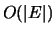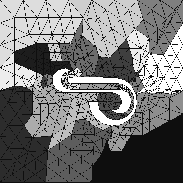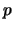Next: Other Partitioning Algorithms Up: Graph Theory Based Algorithms Previous: Spectral bisection

### K-L algorithm

The K-L (Kernighnan-Lin) algorithm [53,24,69] was first suggested in 1970 for bisecting graphs in relation to VLSI layout. It is an iterative algorithm. Starting from a load balanced initial bisection, it first calculates for each vertex the gain in the reduction of edge-cut that may result if that vertex is moved from one partition of the graph to the other. At each inner iteration, it moves the unlocked vertex which has the highest gain, from the partition in surplus (that is, the partition with more vertices) to the partition in deficit. This vertex is then locked and the gains updated. The procedure is repeated even if the highest gain may be negative, until all of the vertices are locked. The last few moves that had negative gains are then undone and the bisection is reverted to the one with the smallest edge-cut so far in this iteration. This completes one outer iteration of the K-L algorithm and the iterative procedure is restarted. Should an outer iteration fail to result in any reductions in the edge-cut or load imbalance, the algorithm is terminated. The initial bisection is generated randomly and for large graphs, the final result is very dependent on the initial choice. The K-L algorithm is a local optimization algorithm, with a limited capability for getting out of local minima by way of allowing moves with negative gain.

By using appropriate data structures, it is possible to implement the K-L algorithm so that each outer iteration has a complexity of. Figure 8 shows the best result among 3 runs of the algorithm on the mesh with 788 elements, it has 133 inter boundary edges.The K-L algorithm is a 2-way local refinement algorithm. It is able to reduce the edge-cut of an existing bisection and can be combined with any of the other partitioning algorithms. It has been extended to-way refinement (see Section 3.4).Next: Other Partitioning Algorithms Up: Graph Theory Based Algorithms Previous: Spectral bisection

2000-03-21